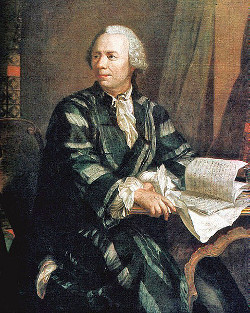# An Odd FactMentioned in James Tanton’s Mathematics Galore!:

In 1740 the French mathematician Philippe Naudé sent a letter to Leonhard Euler asking how many ways a positive integer could be written as a sum of distinct positive integers (regardless of their order). In considering the problem Euler found something remarkable.

Let D(n) be the number of ways to write n as a sum of distinct positive integers. So, for example, D(6) is 4 because there are four ways to do this for 6: 6, 5 + 1, 4 + 2, and 3 + 2 + 1.

And let O(n) be the number of ways to write n as a sum of odd integers. So O(6) is 4 because 6 can be written as 5 + 1, 3 + 3, 3 + 1 + 1 + 1, or 1 + 1 + 1 + 1 + 1 + 1.

Euler showed that O(N) always equals D(N).